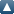# Browse by PersonUp a level
 Export as ASCII CitationBibTeXDublin CoreEndNoteHTML CitationMETSObject IDsOpenURL ContextObjectRDF+N-TriplesRDF+N3RDF+XMLReferReference Manager
Group by: Item Type | No Grouping
Number of items: 23.

## Article

Ohkitani, K. (2018) Study of the 3D Euler equations using Clebsch potentials: dual mechanisms for geometric depletion (vol 31, pg R25, 2018). Nonlinearity, 31 (8). p. 3973. ISSN 0951-7715

Vanon, R. and Ohkitani, K. (2018) Applications of a Cole-Hopf transform to the 3D Navier-Stokes equations. Journal of Turbulence. ISSN 1468-5248

Vanon, R. and Ohkitani, K. (2018) Applications of a Cole-Hopf transform to the 3D Navier-Stokes equations. Journal of Turbulence, 19 (4). ISSN 1468-5248

Ohkitani, K. (2018) Study of the 3D Euler equations using Clebsch potentials: dual mechanisms for geometric depletion. Nonlinearity, 31 (2). ISSN 0951-7715

Ohkitani, K. (2017) Cole-Hopf--Feynman-Kac formula and quasi-invariance of Navier-Stokes equations. Journal of Physics A: Mathematical and Theoretical, 50 (40). ISSN 1751-8113

Ohkitani, K. (2017) Analogue of the Cole-Hopf transform for the incompressible Navier-Stokes equations and its application. Journal of Turbulence.

Ohkitani, K. (2017) Characterization of blowup for the Navier-Stokes equations using vector potentials. AIP Advances, 7 (1). 015211.

Ohkitani, K. (2017) Near-invariance under dynamic scaling for the Navier-Stokes equations in critical spaces: a probabilistic approach to regularity problems. Journal of Physics A: Mathematical and Theoretical, 50 (4). 5501. ISSN 1751-8113

Ohkitani, K. (2016) Study of the Navier–Stokes regularity problem with critical norms. Fluid Dynamics Research, 48 (2). 021401. ISSN 0169-5983

Ohkitani, K. (2016) Late formation of singularities in solutions to the Navier–Stokes equations. Journal of Physics A: Mathematical and Theoretical, 49 (1). 015502. ISSN 1751-8113

Ohkitani, K. (2015) Dynamical equations for the vector potential and the velocity potential in incompressible irrotational Euler flows: A refined Bernoulli theorem. Physical Review E, 92 (3). 033010. ISSN 1539-3755

Ohkitani, K. (2012) The Fefferman-Stein decomposition for the Constantin-Lax-Majda equation: Regularity criteria for inviscid fluid dynamics revisited. Journal of Mathematical Physics, 53 (11). 115607. ISSN 0022-2488

Dowker, M. and Ohkitani, K. (2012) Intermittency and local Reynolds number in Navier-Stokes turbulence: A cross-over scale in the Caffarelli-Kohn-Nirenberg integral. Physics of Fluids, 24 (11). 115112. ISSN 1070-6631

Ohkitani, K. (2012) Asymptotics and numerics of a family of two-dimensional generalized surface quasi-geostrophic equations. Physics of Fluids, 24 (9). 095101. ISSN 1070-6631

Ohkitani, K. and Dowker, M. (2012) Numerical study on comparison of Navier-Stokes and Burgers equations. Physics of Fluids, 24 (5). 055113. ISSN 1070-6631

Ohkitani, K. (2011) Growth rate analysis of scalar gradients in generalized surface quasigeostrophic equations of ideal fluids. Physical Review E, 83 (3). 036317. ISSN 1539-3755

Ohkitani, K. (2010) Numerical study on the incompressible Euler equations as a Hamiltonian system: Sectional curvature and Jacobi field. Physics of Fluids, 22 (5). Art no.057101 . ISSN 1070-6631

Ohkitani, K. and Dowker, M. (2010) Burgers equation with a passive scalar: Dissipation anomaly and Colombeau calculus. Journal of Mathematical Physics, 51 (3). 033101. ISSN 0022-2488

Ohkitani, K. (2009) Nonlinearity depletion, elementary excitations and impulse formulation in vortex dynamics. Journal of Geophysical & Astrophysical Fluid Dynamics, 103 (2&3). pp. 113-133. ISSN 0309-1929

Ohkitani, K. and Constantin, P. (2008) Two- and three-dimensional magnetic reconnection observed in the Eulerian-Lagrangian analysis of magnetohydrodynamics equations. Physical Review E , 78 (6). Art. No. 066315. ISSN 1550-2376

Ohkitani, K. (2008) A miscellany of basic issues on incompressible fluid equations. Nonlinearity, 21. T255-T271. ISSN 0951-7715

Yamada, M. and Ohkitani, K. (1998) Asymptotic formulas for the Lyapunov spectrum of fully developed shell model turbulence. Physical Review E, 57 (6). R6257-R6260. ISSN 1539-3755

## Book Section

Ohkitani, K. (2018) Quasi-invariance for the Navier-Stokes equations. In: Fefferman, C.L., Robinson, J.C. and Rodrigo, J.L., (eds.) Partial Differential Equations in Fluid Mechanics. London Mathematical Society Lecture Note Series, 452 . Cambridge University Press , Cambridge , pp. 97-112. ISBN 9781108460965

This list was generated on Sun Nov 24 20:18:24 2019 GMT.